Search

Systems of Non-Linear Equations:
Solving Simple Systems
(page 3 of 6)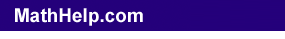To find the exact solution to a system of equations, you must use algebra. Let's look at that first system again:

• Solve the following system algebraically:
• y = x2
y
= 8  x2

Since I am looking for the intersection points, I am therefore looking for the points where the equations overlap, where they share the same values. That is, I am trying to find any spots where y = x2 equals y = 8  x2:

y = x2 = y = 8  x2

The algebra comes in when I manipulate useful bits of this last equation. I can pick out whichever parts I like. (They're all equal, after all -- at least at the intersection points, but the intersection points are the only points that I care about anyway!) So I can pick out any of the following:

y = x2
y = 8  x2
y = y
x
2 = 8  x2

Each of these sub-equations is true, but only the last one is usefully new and different:

x2 = 8  x2

I can solve this for the x-values that make the equation true:

x2 = 8  x2
2x2 = 8
x2 = 4
x = 2, +2

Then the solutions to the original system will occur when x = 2 and when x = +2.

 Advertisement

What are the corresponding y-values? To find them, I plug the x-values back in to either of the two original equations. (It doesn't matter which one I pick because I only care about the points where the equations spit out the same values. So I can pick whichever equation I like better.) I'll plug the x-values into the first equation, because it's the simpler of the two:

x = 2:

y = x2
y = (2)2 = 4

x = +2:

y = x2
y = (+2)2 = 4

Then the solutions (as we already knew) are (x, y) = (2, 4) and (2, 4).

In the above example, the solutions were nice, "neat" integer values; no fractions or decimals. But solutions will not always be neat, so, while the pictures can be very useful for giving you a "feel" for what is going on, graphing is not as accurate as doing the algebra.

Warning: Students are often taught nowadays to "round" absolutely everything, and are thus implicitly taught that all answers will be "neat" answers. But this is wrong; don't fall for it!

For instance:

• Solve the following system:
• y = x2 + 3x + 2
y = 2x + 3

I can solve this in the same manner as we did on the previous problem. The "solution" to the system will be any point(s) that the lines share; that is, any point(s) where the x-value and corresponding y-value for y = x2 + 3x + 2 is the same as the x-value and corresponding y-value for y = 2x + 3; that is, where the lines overlap or intersect; that is, where y = x2 + 3x + 2 equals y = 2x + 3.  Copyright © 2002-2011 Elizabeth Stapel All Rights Reserved

 Looking at the graph of the system: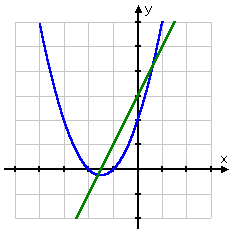...I can see that there appear to be solutions at around (x, y) = (1.5, 0.25) and (x, y) = (0.5, 4.25).  But I cannot assume that this is the answer!  The picture can give me a good idea, but only the algebra can give me the actual answer. I'll set the right-hand sides of the two equations equal to each other, and solve: x2 + 3x + 2 = 2x + 3x2 + x  1 = 0

Content Continues Below

 Using the Quadratic Formula gives me: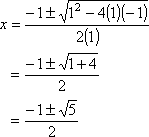Then I have one solution: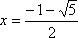...which has a corresponding  y-value of: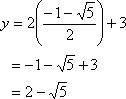The other solution (from the "±" in front of the square root) is: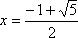....which gives me a y-value of: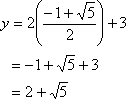So the solutions are: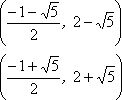For purposes of graphing, the approximate solutions are:

(x, y) = (1.62, 0.24) and (0.62, 4.24).

In other words, while my guess from the picture was close, it was not entirely correct nor "exact".

(Note: If the algebra had given me answers that are far afield of these picture-based guesses, I would have been able to safely assume that I had messed up the math somewhere. In this way, the graph can be very helpful for checking your work.)

<< Previous  Top  |  1 | 2 | 3 | 4 | 5 | 6  |  Return to Index  Next >>

 Cite this article as: Stapel, Elizabeth. "Systems of Non-Linear Equations: Solving Simple Systems." Purplemath.     Available from https://www.purplemath.com/modules/syseqgen3.htm.     Accessed [Date] [Month] 2016

 Copyright © 2021  Elizabeth Stapel   |   About   |   Terms of Use   |   Linking   |   Site Licensing Contact Us